# Test Prep Plan - Take a practice test

Take this practice test to check your existing knowledge of the course material. We'll review your answers and create a Test Prep Plan for you based on your results.
How Test Prep Plans work
1
2Based on your results, we'll create a customized Test Prep Plan just for you!
3Study smarter
Study more effectively: skip concepts you already know and focus on what you still need to learn.

# WEST Middle Grades Math: Linear Equations Chapter Exam

Exam Instructions:

Choose your answers to the questions and click 'Next' to see the next set of questions. You can skip questions if you would like and come back to them later with the yellow "Go To First Skipped Question" button. When you have completed the practice exam, a green submit button will appear. Click it to see your results. Good luck!

### Page 1

#### Question 2 2. Which linear equation is shown below?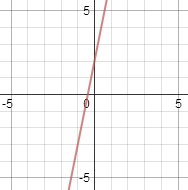#### Question 3 3. What is the slope-intercept form equation of the graph below?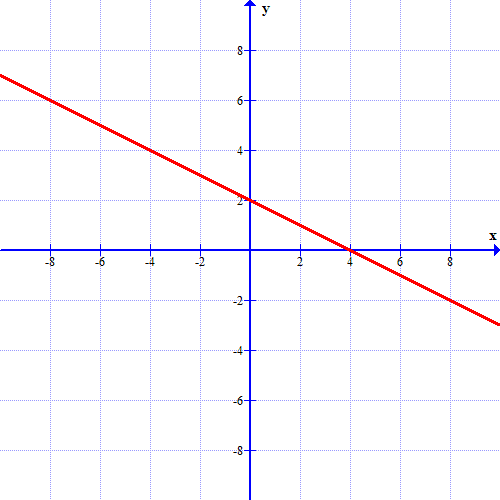### Page 3

#### Question 13 13. If the graph below was written in the slope-intercept form, which of the following would be true?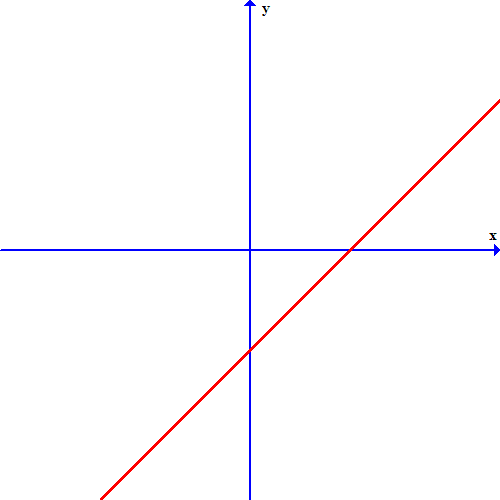### Page 4

#### Question 17 17. Which one of the following equations could work for the following graph?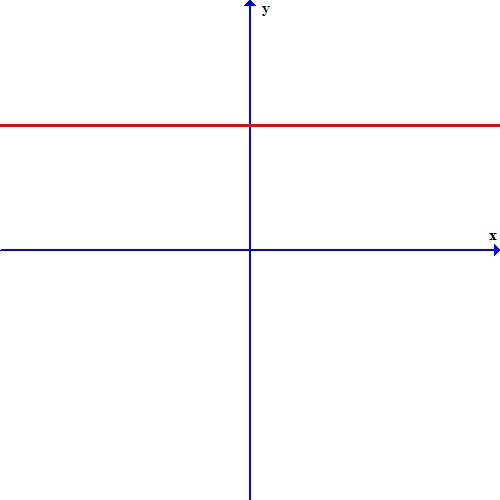#### Question 20 20. What is the equation of the graph below?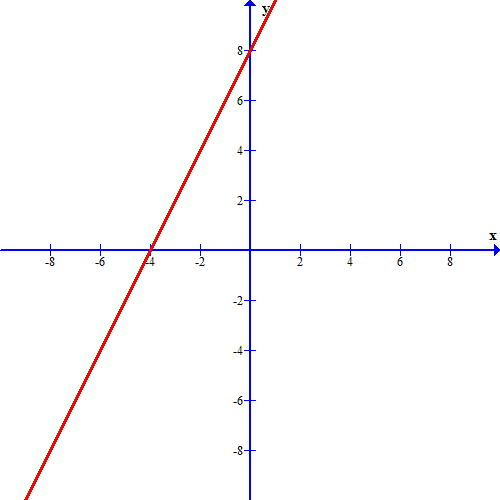### Page 5

#### Question 21 21. Which of these equations represents a line that is perpendicular to the line in the graph below?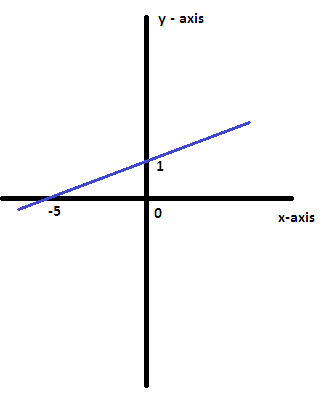#### Question 22 22. If the graph below has the equation Ax+By=C, which statement MUST be true?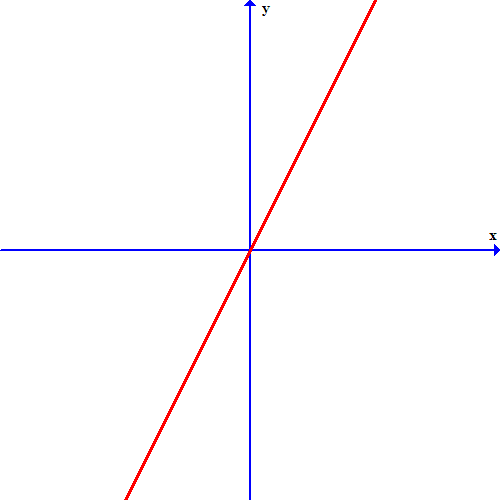#### Question 23 23. If the graph below has the equation Ax + By = -4, what must be true about A and B?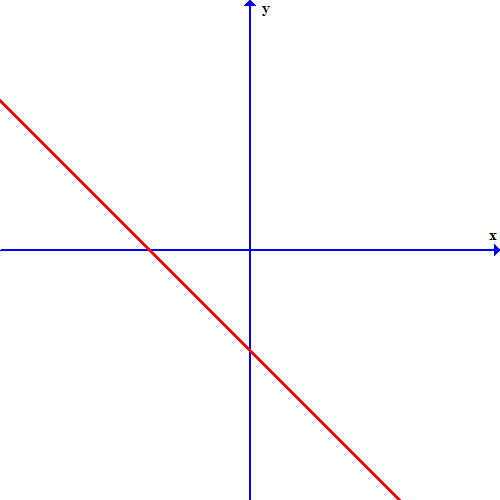#### WEST Middle Grades Math: Linear Equations Chapter Exam Instructions

Choose your answers to the questions and click 'Next' to see the next set of questions. You can skip questions if you would like and come back to them later with the yellow "Go To First Skipped Question" button. When you have completed the practice exam, a green submit button will appear. Click it to see your results. Good luck!

Support